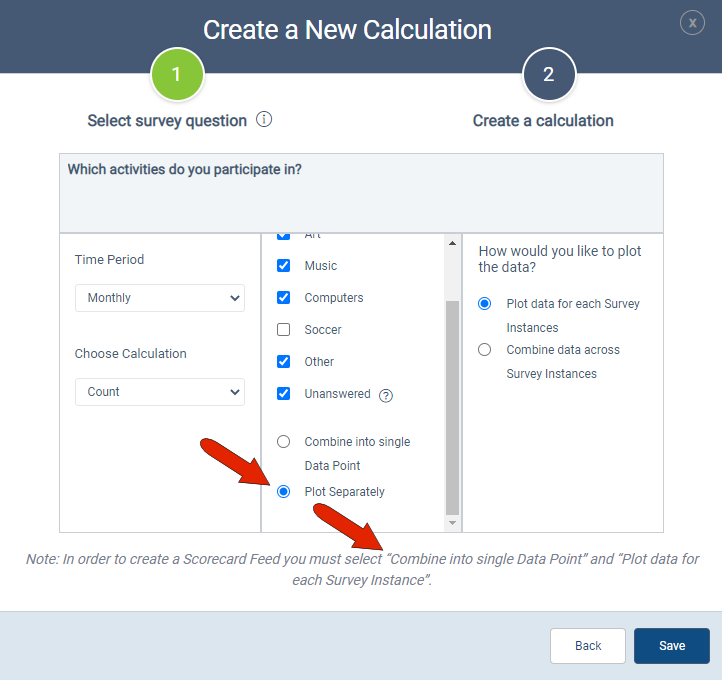# Types of Calculations

The types of calculations available depend on the format of the survey question

 Field type Count Percent of Total Sum Average Max Min StdDev Custom Single Choice (Radio Buttons) X X -- -- -- -- -- -- Single Choice (Radio Buttons) with Numeric Values X -- X X X X X X Multiple Choice (Check Boxes) X X -- -- -- -- -- -- Multiple Choice (Check Boxes) with Numeric Values X -- X X X X X X Single Choice (Dropdown) X X -- -- -- -- -- -- Single Choice (Dropdown) with Numeric Values X -- X X X X X X Multiple Choice (Multiselect) X X -- -- -- -- -- -- Multiple Choice (Multiselect) with Numeric Values X -- X X X X X X Number X -- X X X X X X Range X -- X X X X X X Date X -- -- -- -- -- -- -- True/False X -- X X X X X X True/False with Numeric Values X -- X X X X X X Time X -- -*- -*- X X --* --* Custom Calculation (Sum All) X -- X X X X X X Custom Calculation (Avg All) X -- X X X X X X Custom Calculation (Min All) X -- X X X X X X Custom Calculation (Max All) X -- X X X X X X Custom Calculation (Custom) X -- X X X X X X Participant Field (acts as text) X -- -- -- -- -- -- --

In this example, the survey question has a multiple check box format. So calculations can be only Count or Percentage of Total, and you can choose which answer choices to include. Including “Unanswered” means that responses will be counted from participants who skipped this question. You would see similar options for single-choice responses.Because this type of question has multiple answers, you also have the option of combining all answers into a single data point, or plotting each answer separately. Separated data provides more detailed results, but you won’t be able to create a scorecard feed. With separated data, you also cannot segment the data.